smoothness

Smoothness of path

Description

example

smoothness(pathMetricsObj) evaluates the smoothness of the planned path. Values close to 0 indicate a smoother path. Straight-line paths return a value of 0.

smoothness(pathMetricsObj,'Type','segments') returns individual smoothness calculations between each set of three poses on the path, in the form of a (n–2)-element vector, where n is the number of poses.

Examples

collapse all

Compute smoothness, clearance, and validity of a planned path based on a set of poses and the associated map environment.

Load and Assign Map to State Validator

Create an occupancy map from an example map and set the map resolution.

mapResolution = 1; % cells/meter
map = occupancyMap(simpleMap,mapResolution);

Create a Dubins state space.

statespace = stateSpaceDubins;

Create a state validator based on occupancy map to store the parameters and states in the Dubins state space.

statevalidator = validatorOccupancyMap(statespace);

Assign the map to the validator.

statevalidator.Map = map;

Set the validation distance for the validator.

statevalidator.ValidationDistance = 0.01;

Update the state space bounds to be the same as the map limits.

statespace.StateBounds = [map.XWorldLimits;map.YWorldLimits;[-pi pi]];

Plan Path

Create an RRT* path planner and allow further optimization.

planner = plannerRRTStar(statespace,statevalidator);
planner.ContinueAfterGoalReached = true;

Reduce the maximum number of iterations and increase the maximum connection distance.

planner.MaxIterations = 2500;
planner.MaxConnectionDistance = 0.3;

Define start and goal states for the path planner as [x, y, theta] vectors. x and y are the Cartesian coordinates, and theta is the orientation angle.

start = [2.5, 2.5, 0]; % [meters, meters, radians]
goal = [22.5, 8.75, 0];

Plan a path from the start state to the goal state. The plan function returns a navPath object.

rng(100,'twister') % repeatable result
[path,solutionInfo] = plan(planner,start,goal);

Compute and Visualize Path Metrics

Create a path metrics object.

pathMetricsObj = pathmetrics(path,statevalidator);

Check path validity. The result is 1 (true) if the planned path is obstacle free. 0 (false) indicates an invalid path.

isPathValid(pathMetricsObj)
ans = logical
1

Calculate the minimum clearance of the path.

clearance(pathMetricsObj)
ans = 1.4142

Evaluate the smoothness of the path. Values close to 0 indicate a smoother path. Straight-line paths return a value of 0.

smoothness(pathMetricsObj)
ans = 1.7318

Visualize the minimum clearance of the path.

show(pathMetricsObj)
legend('Planned Path','Minimum Clearance')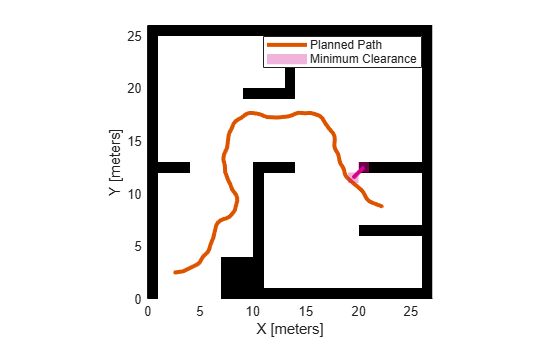Plan a vehicle path through a parking lot using the RRT* algorithm. Compute and visualize the smoothness, clearance, and validity of the planned path.

Load and Assign Map to State Validator

Load a costmap of a parking lot. Plot the costmap to see the parking lot and the inflated areas that the vehicle should avoid.

costmap = parkingLotCostmap;
plot(costmap)
xlabel('X (meters)')
ylabel('Y (meters)')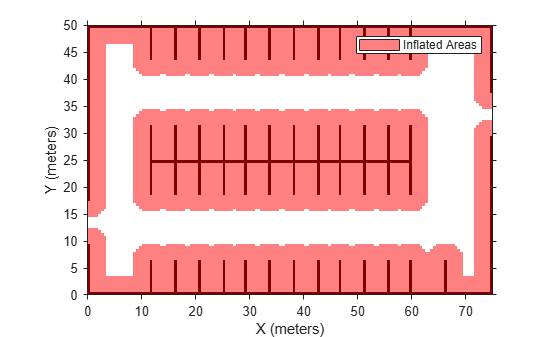Create a stateSpaceDubins object and increase the minimum turing radius to 4 meters.

statespace = stateSpaceDubins;

Create a validatorVehicleCostmap object using the created state space.

statevalidator = validatorVehicleCostmap(statespace);

Assign the parking lot costmap to the state validator object.

statevalidator.Map = costmap;

Plan Path

Define start and goal poses for the vehicle as [x, y, Θ] vectors. World units for the (x,y) locations are in meters. World units for the orientation angles Θ are in degrees.

startPose = [5, 5, 90]; % [meters, meters, degrees]
goalPose = [40, 38, 180]; % [meters, meters, degrees]

Use a pathPlannerRRT (Automated Driving Toolbox) object and the plan (Automated Driving Toolbox) function to plan the vehicle path from the start pose to the goal pose.

planner = pathPlannerRRT(costmap);
refPath = plan(planner,startPose,goalPose);

Interpolate along the path at every one meter. Convert the orientation angles from degrees to radians.

poses = zeros(size(refPath.PathSegments,2)+1,3);
poses(1,:) = refPath.StartPose;
for i = 1:size(refPath.PathSegments,2)
poses(i+1,:) = refPath.PathSegments(i).GoalPose;
end

Create a navPath object using the Dubins state space object and the states specified by poses.

path = navPath(statespace,poses);

Compute and Visualize Path Metrics

Create a pathmetrics object.

pathMetricsObj = pathmetrics(path,statevalidator);

Check path validity. The result is 1 (true) if the planned path is obstacle free. 0 (false) indicates an invalid path.

isPathValid(pathMetricsObj)
ans = logical
1

Compute and visualize the minimum clearance of the path.

clearance(pathMetricsObj)
ans = 0.5000
show(pathMetricsObj)
legend('Inflated Areas','Planned Path','Minimum Clearance')
xlabel('X (meters)')
ylabel('Y (meters)')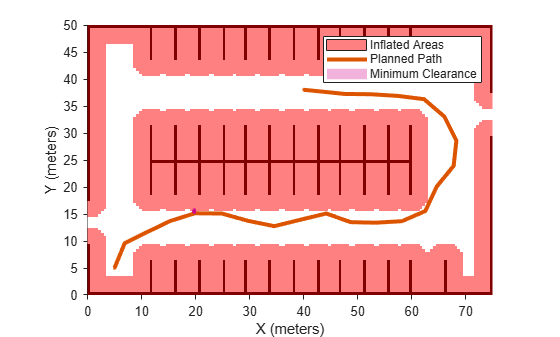Compute and visualize the smoothness of the path. Values close to 0 indicate a smoother path. Straight-line paths return a value of 0.

smoothness(pathMetricsObj)
ans = 0.0842
show(pathMetricsObj,'Metrics',{'Smoothness'})
legend('Inflated Areas','Path Smoothness')
xlabel('X (meters)')
ylabel('Y (meters)')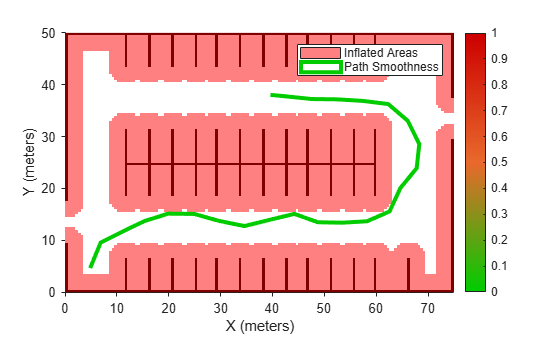Visualize the clearance for each state of the path.

show(pathMetricsObj,'Metrics',{'StatesClearance'})
legend('Inflated Areas','Planned Path','Clearance of Path States')
xlabel('X (meters)')
ylabel('Y (meters)')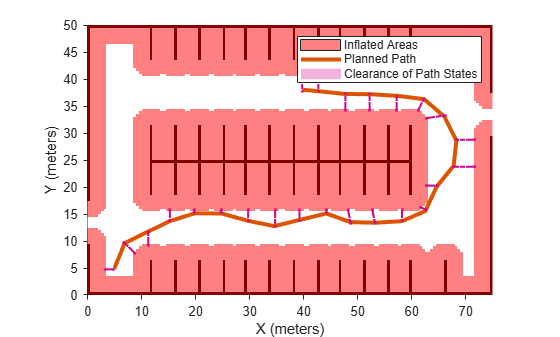Input Arguments

collapse all

Information for path metrics, specified as a pathmetrics object.

 Lindemann, Stephen R., and Steven M. LaValle. "Simple and efficient algorithms for computing smooth, collision-free feedback laws over given cell decompositions." The International Journal of Robotics Research 28, no. 5. 2009, pp. 600-621.